# 1. Python Diffraction-Interference module## 1.1. Features

Diffractio is a Python library for Diffraction and Interference Optics.

It implements Scalar and vector Optics. The main algorithms used are:

• Fast Fourier Transform (FFT).
• Rayleigh Sommerfeld (RS).
• Chirp z-transform (CZT).
• Plane Wave Descomposition (PWD).
• Beam Propagation Method (BPM).
• Wave Propagation Method (WPM).
• Vectorial Rayleigh-Sommerfeld (VRS).
• Vector Fast Fourier Tranform (VFFT).
• Vector Chirp z-transform (VCZT).

When possible, multiprocessing is implemented for a faster computation.

The scalar propagation schemes are implemented in modules:

• X - fields are defined in the x axis.
• Z - fields are defined in the z axis (for visualiztion and analysis).
• XZ - fields are defined in the xz plane, being z the propagation direction.
• XY - fields are defined in the xy transversal plane.
• XYZ - fields are defined in the xyz volume.

Each scheme present three modules:

• sources: Generation of light.
• fields: Propagation techniques, parameters and general functions.

The vector propagation schemes are implemented in modules:

• vector_XY - Ex, Ey, and Ez electric field components are defined, which allows polarization analysis.

There are additional schemes used mainly for representation.

• vector_X - fields are defined in the z axis (for visualiztion and analysis).
• vector_Z - fields are defined in the z axis (for visualiztion and analysis).
• vector_XZ - fields are defined in the xz axis (for visualiztion and analysis).
• vector_XYZ - fields are defined in the xyz axis (for visualiztion and analysis).

For the vector analysis, we also use the py_pol module: https://pypi.org/project/py-pol/

### 1.1.1. Sources

One main part of this software is the generation of optical fields such as:

• Plane waves.
• Spherical waves.
• Gaussian beams.
• Bessel beams.
• Vortex beams.
• Laguerre beams.
• Hermite-Gauss beams.
• Zernike beams.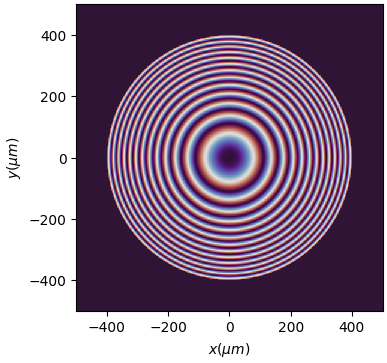Another important part of Diffractio is the generation of masks and Diffractive Optical Elements such as:

• Slits, double slits, circle, square, ring …
• Lenses, diffractive lenses, aspherical lenses…
• Axicon, prisms, biprism, image, rough surface, gray scale …
• Gratings: Ronchi, phase, radial, angular, sine, forked, blazed, 2D, chess…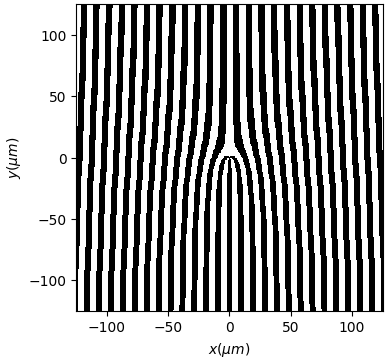In the XZ scheme there is also a number of masks:

• image, sphere, layer, lenses, wedge, prism, probe, gratings…
• Surfaces can be added to objects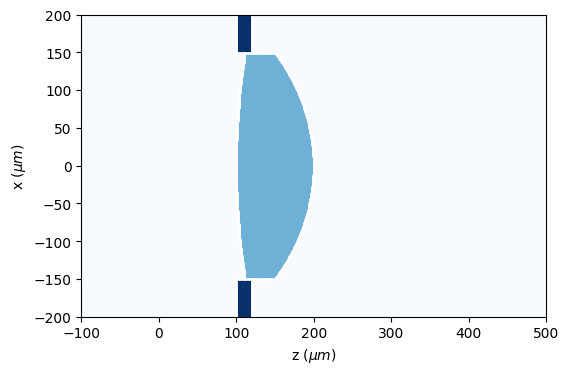### 1.1.3. Fields

Several propagation algorithms for propagation of light are implemented in the fields modules:

• Fast Fourier Transform (FFT) which allows, in a single step, to determine the field at the far field.

• Rayleigh-Sommerfeld (RS) [Appl. Opt., 45(6) 1102–1110, (2006)] RS allows, in a single step, to propagate to a near or far observation plane, which allows fast computations. The fields and the masks must be defined in a plane.

• Beam propagation method (BPM) [Appl. Opt. 24, 3390-3998 (1978)] which allows to analyze the propation of light in volumetric elements, such as spheres, cylinders and other complex forms, provided that the spatial variations in the refraction index are small. It allows graded index structures. It presents a complexity of O(n) in the two-dimensional and O(n2) in the three-dimensional case. It is computed according to the split-step propagation scheme.

• Wave Propagation Method (WPM). [Appl. Opt. 32, 4984 (1993)] WPM was introduced in order to overcome the major limitations of the beam propagation method (BPM). With the WPM, the range of application can be extended from the simulation of waveguides to simulation of other optical elements like lenses, prisms and gratings. WPM can accurately simulate scalar light propagation in inhomogeneous media at high numerical apertures, and provides valid results for propagation angles up to 85° and that it is not limited to small index variations in the axis of propagation. Fast implementation with discrete number of refraction indexes is also implemented.

• Chirped Z-Transform (CZT). [Light: Science and Applications, 9(1), (2020)] CZT allows, in a single step, to propagate to a near or far observation plane. It present advantages with respecto to RS algorithm, since the region of interest and the sampling numbers can be arbitrarily chosen, endowing the proposed method with superior flexibility. CZT algorithm allows to have a XY mask and compute in XY, Z, XZ, XYZ schemes, simply defining the output arrays.

• Vector Rayleigh-Sommerfeld (VRS). The VRS method [Laser Phys. Lett. 10(6) 065004 (2013)] allows to propagate (Ex,Ey,Ez) fields offering the advantage of significant reduction in computation, from flat diffractive elements (Thin Element Approximation) with full control of polarization. It addresses simultaneously both longitudinal polarization. This approach offers the advantage of significant reduction in computation.

• Vector Chirped Z-Transform (VCZT). [Light: Science and Applications, 9(1), (2020)]. CZT is also implemented in vector fields.

The fields, masks and sources can be stored in files.

Also drawings can be easily obtained, for intensity, phase, fields, etc.

In some modules, videos can be generated for a better analysis of optical fields.

### 1.1.4. Vector beams

Here, we implement new classes where the E_x, E_y, and E_z fields are generated and propagated using Rayleigh-Sommerfeld and Chirped z-transform algorithms. Also, simple and complex polarizing masks can be created.

Intensity of vector field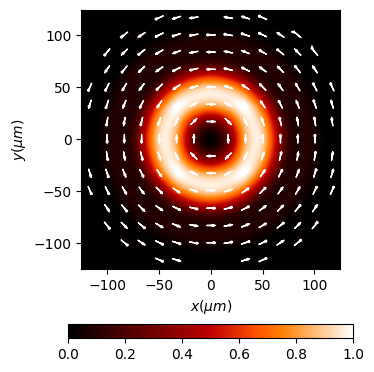Polarization: Stokes parameters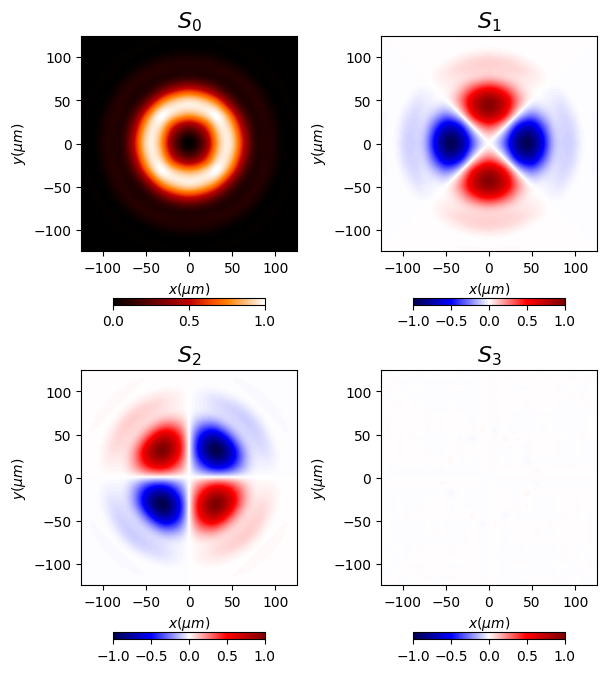### 1.1.5. Other features

• Intensity, MTF and other parameters are obtained from the optical fields.
• Fields can be added simply with the + signe, and interference is produced. Masks can be multiplied, added and substracted in order to make complex structures
• Resampling fields in order to analyze only areas of interest.
• Save and load data for future analysis.
• Rayleigh-Sommerfeld implementation is performed in multiprocessing for fast computation.
• Polychromatic and extended source problems can also be analyzed using multiprocessing.

## 1.2. Authors

• Luis Miguel Sanchez Brea <optbrea@ucm.es>

Universidad Complutense de Madrid, Faculty of Physical Sciences, Department of Optics Plaza de las ciencias 1, ES-28040 Madrid (Spain)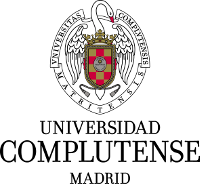## 1.3. Citing

L.M. Sanchez Brea, “Diffractio, python module for diffraction and interference optics”, https://pypi.org/project/diffractio/ (2019)

## 1.4. References for algorithms

RS

• Shen, F. & Wang, A. “Fast-Fourier-transform based numerical integration method for the Rayleigh-Sommerfeld diffraction formula. Appl. Opt. 45, 1102–1110 (2006).

WPM

• K.-H. Brenner, W. Singer, “Light propagation through micro lenses: a new simulation method”, Appl. Opt., 32(6) 4984-4988 (1993).
• Schmidt, S. et al. “Wave-optical modeling beyond the thin-element-approximation” Opt. Express 24, 30188 (2016).
• Brenner, K.H. “A high-speed version of the wave propagation method applied to micro-optics.” 16th Workshop on Information Optics (WIO). IEEE (2017)
• Schmidt, S., et al. “Rotationally symmetric formulation of the wave propagation method-application to the straylight analysis of diffractive lenses” Opt. Lett. 42, 1612 (2017).

VRS

• Ye, H. et al. “Creation of a longitudinally polarized subwavelength hotspot with an ultra-thin planar lens: Vectorial Rayleigh-Sommerfeld method” Laser Phys. Lett. 10, (2013).

CZT

• Bluestein, L., “A linear filtering approach to the computation of the discrete Fourier transform,” Northeast Electronics Research and Engineering Meeting Record 10, 218-219 (1968).
• Hu Y. et al. “Efficient full-path optical calculation of scalar and vector diffraction using the Bluestein method” Light: Science & Applications 9(119) (2020)

VCZT

• Leutenegger M. et al. “Fast focus field calculations” Optics Express 14(23) 11277 (2006).
• Hu Y. et al. “Efficient full-path optical calculation of scalar and vector diffraction using the Bluestein method” Light: Science & Applications 9(119) (2020)

VWPM (not implemented yet)

• Fertig, M., Brenner, K.-H. “Vector wave propagation method”. J. Opt. Soc. Am. A 27, 709 (2010).
• Fertig, M., Brenner, K.-H. “Vector wave propagation method”. PhD thesis (Mannheim, 2010).

## 1.5. Other References

• J.W. Goodman, “Introduction to Fourier Optics” McGraw-Hill, 1996.
• B.E. Saleh y M. C. Teich, “Fundamentals of photonics” John Wiley & Sons, 2019.
• Z.Qiwen, “Vectorial optical fields: Fundamentals and applications” World scientific, 2013.
• “Numerical Methods in Photonics Lecture Notes”. http://ecee.colorado.edu/~mcleod/teaching/nmip/lecturenotes.html.